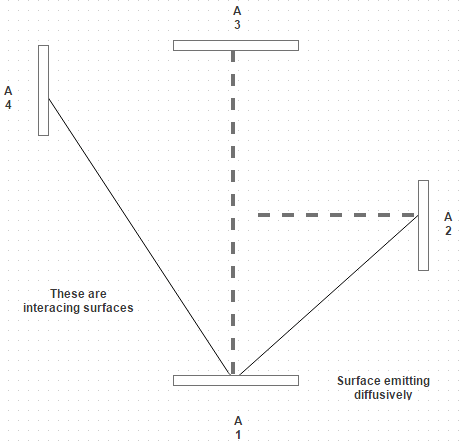Test: Intensity of Radiations

# Test: Intensity of Radiations

Test Description

## 10 Questions MCQ Test Heat Transfer | Test: Intensity of Radiations

Test: Intensity of Radiations for Chemical Engineering 2022 is part of Heat Transfer preparation. The Test: Intensity of Radiations questions and answers have been prepared according to the Chemical Engineering exam syllabus.The Test: Intensity of Radiations MCQs are made for Chemical Engineering 2022 Exam. Find important definitions, questions, notes, meanings, examples, exercises, MCQs and online tests for Test: Intensity of Radiations below.
Solutions of Test: Intensity of Radiations questions in English are available as part of our Heat Transfer for Chemical Engineering & Test: Intensity of Radiations solutions in Hindi for Heat Transfer course. Download more important topics, notes, lectures and mock test series for Chemical Engineering Exam by signing up for free. Attempt Test: Intensity of Radiations | 10 questions in 10 minutes | Mock test for Chemical Engineering preparation | Free important questions MCQ to study Heat Transfer for Chemical Engineering Exam | Download free PDF with solutions
 1 Crore+ students have signed up on EduRev. Have you?
Test: Intensity of Radiations - Question 1

### The solid angle is defined by a region by the rays of a sphere, and is measured as Where, An is projection of incident surface normal to line of propagation.

Detailed Solution for Test: Intensity of Radiations - Question 1

Solid angle is represented by α.

Test: Intensity of Radiations - Question 2

### The plane angle is defined by a region by the rays of a circle, and is measured as\ Where, L is arc of length and r is radius of circle.

Detailed Solution for Test: Intensity of Radiations - Question 2

It is the ratio of arc of length on the circle to the radius of the circle.

Test: Intensity of Radiations - Question 3

### When the incident surface is a sphere, the projection of surface normal to the line of propagation is the silhouette disk of the sphere which is a circle of the diameter of

Detailed Solution for Test: Intensity of Radiations - Question 3

It must be a circle of diameter of a sphere.

Test: Intensity of Radiations - Question 4

If I denotes the normal intensity and I α represents the intensity at angle α, then

Detailed Solution for Test: Intensity of Radiations - Question 4

The intensity of radiation in a direction from the normal is proportional to cosine of the angle.

Test: Intensity of Radiations - Question 5

The intensity of normal radiation I n is how much times the emissive power?

Detailed Solution for Test: Intensity of Radiations - Question 5

= σ T 4/ π and E = σ T 4.

Test: Intensity of Radiations - Question 6

A small surface emits diffusively, and measurements indicate that the total intensity associated with emission in the normal direction I = 6500 W/square m sr. The emitted radiation is intercepted by three surfaces. Mark calculations for intensity associated with emissionDetailed Solution for Test: Intensity of Radiations - Question 6

For a diffusion emitter, the intensity of the emitted radiation is independent of direction.

Test: Intensity of Radiations - Question 7

Consider a deep-space probe constructed as 1 m diameter polished aluminum sphere. Estimate the equilibrium temperature that the probe reaches if the solar energy received is 300 W/m2. For solar radiation, absorptivity of aluminum is 0.3 and the average emissivity appropriate for aluminum at low temperature is 0.04

Detailed Solution for Test: Intensity of Radiations - Question 7

in = α q A P = 70.7 W. Q out = E σ A T 4.

Test: Intensity of Radiations - Question 8

The total emissive power of the emitter with area d A and temperature T is given by

Detailed Solution for Test: Intensity of Radiations - Question 8

E = I π d A.

Test: Intensity of Radiations - Question 9

A black body of 0.2 m2 area has an effective temperature of 800 K. Calculate the intensity of normal radiations

Detailed Solution for Test: Intensity of Radiations - Question 9

I= α T 4/π = 7396.28 W/m2 sr.

Test: Intensity of Radiations - Question 10

The energy radiated out decreases with increases in α and becomes zero at an angle of

Detailed Solution for Test: Intensity of Radiations - Question 10

α = I n cos α. So at 90 degree it becomes zero.

## Heat Transfer

58 videos|70 docs|85 tests
 Use Code STAYHOME200 and get INR 200 additional OFF Use Coupon Code
Information about Test: Intensity of Radiations Page
In this test you can find the Exam questions for Test: Intensity of Radiations solved & explained in the simplest way possible. Besides giving Questions and answers for Test: Intensity of Radiations, EduRev gives you an ample number of Online tests for practice

## Heat Transfer

58 videos|70 docs|85 tests